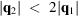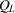International Tables for Crystallography (2006). Vol. B, ch. 4.4, pp. 449-465   | 1 | 2 | https://doi.org/10.1107/97809553602060000566

Contents

• 4.4. Scattering from mesomorphic structures  (pp. 449-465)
• 4.4.1. Introduction  (pp. 449-451) | html | pdf |
• 4.4.2. The nematic phase  (pp. 451-453) | html | pdf |
• 4.4.3. Smectic-A and smectic-C phases  (pp. 453-456) | html | pdf |
• 4.4.3.1. Homogeneous smectic-A and smectic-C phases  (pp. 453-455) | html | pdf |
• 4.4.3.2. Modulated smectic-A and smectic-C phases  (p. 455) | html | pdf |
• 4.4.3.3. Surface effects  (pp. 455-456) | html | pdf |
• 4.4.4. Phases with in-plane order  (pp. 456-463) | html | pdf |
• 4.4.4.1. Hexatic phases in two dimensions  (pp. 457-458) | html | pdf |
• 4.4.4.2. Hexatic phases in three dimensions  (pp. 458-460) | html | pdf |
• 4.4.4.2.1. Hexatic-B  (p. 458) | html | pdf |
• 4.4.4.2.2. Smectic-F, smectic-I  (pp. 458-460) | html | pdf |
• 4.4.4.3. Crystalline phases with molecular rotation  (pp. 460-462) | html | pdf |
• 4.4.4.3.1. Crystal-B  (pp. 460-462) | html | pdf |
• 4.4.4.3.2. Crystal-G, crystal-J  (p. 462) | html | pdf |
• 4.4.4.4. Crystalline phases with herringbone packing  (pp. 462-463) | html | pdf |
• 4.4.4.4.1. Crystal-E  (pp. 462-463) | html | pdf |
• 4.4.4.4.2. Crystal-H, crystal-K  (p. 463) | html | pdf |
• 4.4.5. Discotic phases  (p. 463) | html | pdf |
• 4.4.6. Other phases  (pp. 463-464) | html | pdf |
• 4.4.7. Notes added in proof to first edition  (pp. 464-465) | html | pdf |
• 4.4.7.1. Phases with intermediate molecular tilt: smectic-L, crystalline-M,N  (p. 464) | html | pdf |
• 4.4.7.2. Nematic to smectic-A phase transition  (pp. 464-465) | html | pdf |
• References | html | pdf |
• Figures
• Fig. 4.4.1.1. Illustration of the progression of order throughout the sequence of mesomorphic phases that are based on rod-like' molecules  (p. 449) | html | pdf |
• Fig. 4.4.1.2. Schematic illustration of the real-space molecular order and the scattering cross sections in reciprocal space for the: ( a ) nematic; ( b ) smectic-A; and ( c ), ( d ) smectic-C phases  (p. 450) | html | pdf |
• Fig. 4.4.1.3. Chemical formulae for some of the molecules that form thermotropic liquid crystals  (p. 451) | html | pdf |
• Fig. 4.4.3.1. ( a ) Schematic illustration of the necessary condition for coupling between order parameters when;(p. 455) | html | pdf |
• Fig. 4.4.3.2. Specular reflectivity of ∼8 keV X-rays from the air–liquid interface of the nematic liquid crystal 8OCB 0.05 K above the nematic to smectic-A transition temperature  (p. 456) | html | pdf |
• Fig. 4.4.4.1. ( a ) Schematic illustration of the geometry and ( b ) kinematics of X-ray scattering from a freely suspended smectic film  (p. 457) | html | pdf |
• Fig. 4.4.4.2. Typicalscans from the crystalline-B phases of ( a ) a free film of 7O.7  (p. 457) | html | pdf |
• Fig. 4.4.4.3. Scattering intensities in reciprocal space from two-dimensional: ( a ) liquid; ( b ) crystal; ( c ) normal hexatic; and tilted hexatics  (p. 458) | html | pdf |
• Fig. 4.4.4.4. Scattering intensities in reciprocal space from three-dimensional tilted hexatic phases  (p. 459) | html | pdf |
• Fig. 4.4.4.5. The phase diagram for free films of 7O.7 as a function of thickness and temperature  (p. 459) | html | pdf |
• Fig. 4.4.4.6. Location of the Bragg peaks in one 60° section of reciprocal space for the three-dimensional crystalline-B phases observed in thick films of 7O.7  (p. 461) | html | pdf |
• Fig. 4.4.4.7. ( a ) The herringbone' stacking suggested for the crystalline-E phase in which molecular rotation is partially restricted  (p. 462) | html | pdf |
• Fig. 4.4.5.1. Schematic illustration of the molecular stacking for the discotic ( a )and ( b )phases  (p. 463) | html | pdf |
• Tables
• Table 4.4.1.1. Some of the symmetry properties of the series of three-dimensional phases described in Fig. 4.4.1.1  (p. 449) | html | pdf |
• Table 4.4.1.2. The symmetry properties of the two-dimensional hexatic and crystalline phases  (p. 450) | html | pdf |
• Table 4.4.2.1. Summary of critical exponents from X-ray scattering studies of the nematic to smectic-A phase transition  (p. 453) | html | pdf |